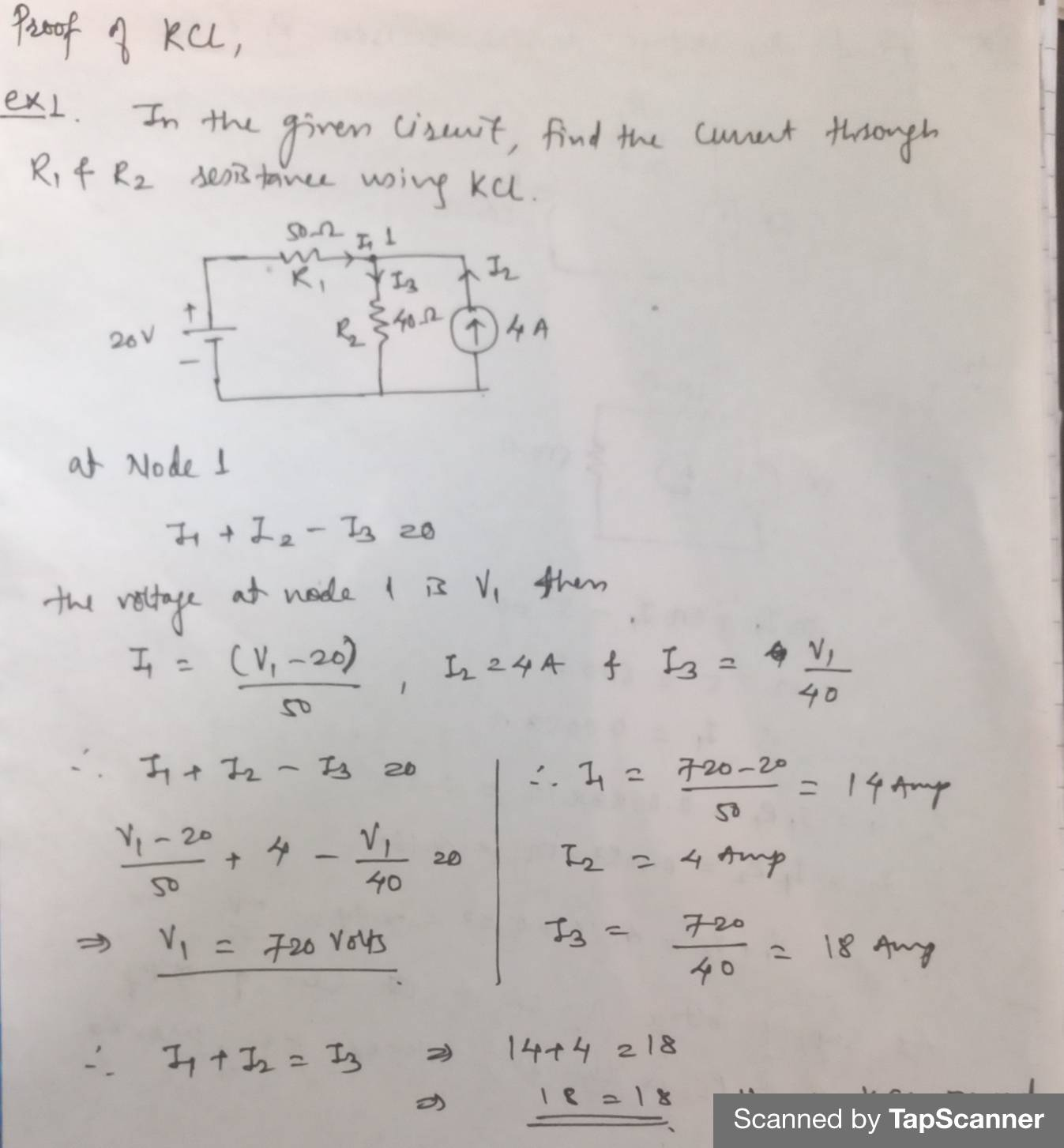### Kirchhof’s Current law

Kirchhof’s Current law:

It states that, “The algebraic sum of all the currents entering into the loop and leaving the loop must be equal to zero.”
Derivation of KCl

Using the equation of continuity,

▽.J + ∂९/∂t = 0
▽.J = -∂९/∂t
∫▽.J dv = -∫∂९/∂t  dv
∮ J.ds = - ∂/∂t ∫९ dv
i = -∂/∂t Q
I.e.   ΣI=0